#### Nondeterministic Dijkstra

Figure 10.8 shows an extension of Dijkstra's algorithm for solving the problem of Formulation 10.1 under nondeterministic uncertainty. It can also be considered as a variant of the algorithm in Figure 10.6 because it grows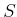by using backprojections. The algorithm in Figure 10.8 represents a backward-search version of Dijkstra's algorithm; therefore, it maintains the worst-case cost-to-go,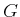, which sometimes becomes the optimal, worst-case cost-to-go,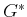. Initially,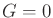for states in the goal, and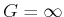for all others.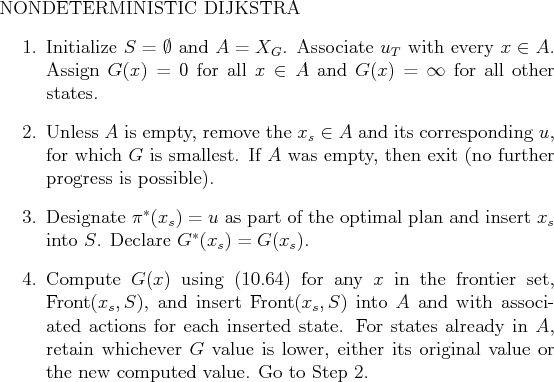Step 1 performs the initialization. Step 2 selects the state in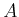that has the smallest value. As in Dijkstra's algorithm for deterministic problems, it is known that the cost-to-go for this state is the smallest possible. It is therefore declared in Step 3 that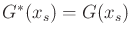, and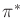is extended to include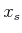.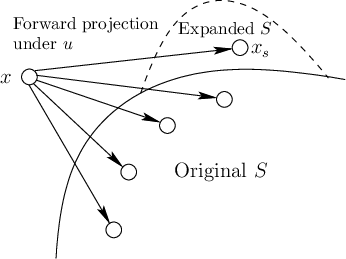Step 4 updates the costs for some states and expands the active set,. Which costs could be immediately affected by the insertion ofinto? These are states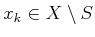for which there exists some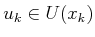that produces a one-stage forward projection,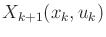, such that: 1)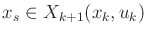and 2)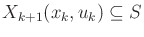. This is depicted in Figure 10.9. Let the set of states that satisfy these constraints be called the frontier set, denoted by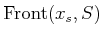. For each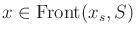, let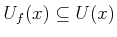denote the set of all actions for which the forward projection satisfies the two previous conditions.

The frontier set can be interpreted in terms of backprojections. The weak backprojection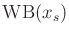yields all states that can possibly reachin one step. However, the cost-to-go is only finite for states in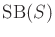(herealready includes). The states inshould certainly be excluded because their optimal costs are already known. These considerations reduce the set of candidate frontier states to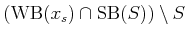. This set is still too large because the same action,, must produce a one-stage forward projection that includesand is a subset of.

The worst-case cost-to-go is computed for allas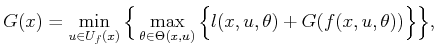(10.63)

in which the restricted action set,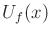, is used. Ifwas already inand a previous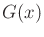was computed, then the minimum of its previous value and (10.64) is kept.

Steven M LaValle 2020-08-14